Next: Structure of the Library Up: Generic programming on automata Previous: Introduction

# Notations

We recall here our definition of an automaton :
We aim to design code with efficiency and genericity in mind, thus we add to the usual abstract type automaton two sets T and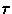in order to associate any kind of information (tags) to the states.
Let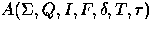be a 7-uple of finite sets defined as follow :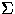the alphabet Q a set of states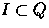a set of initial states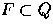a set of final states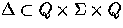a set of transitions T a set of tags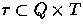a relation between states and tags
Let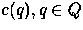be the context of a state :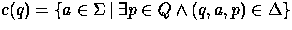, that is the set of the letters on the outgoing edges of a state q.
We define P(X) as the power-set of a set X and |X| as its number of elements.
Let's view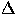as two distinct transition functions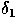and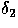according to the most frequent operations needed on an automaton: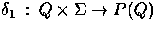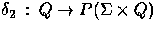These two functions are the corner stone of the library. To put it formally, everything comes down to manipulate a set of triples in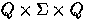and two subsets of Q.

Besides these definitions, we introduce the notion of occupation ratio defined as follow: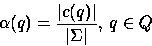and the average value,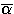as :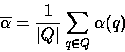This value is crucial in estimating which container fits better when memory space is limited. Intuitively, the closergets to 0, the emptier the transition matrix will be.Next: Structure of the Library Up: Generic programming on automata Previous: Introduction
Vincent Lemaout
12/9/1997